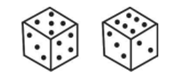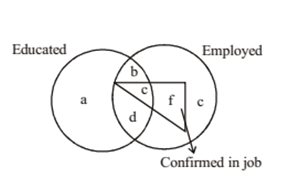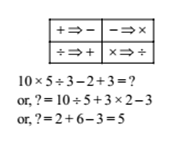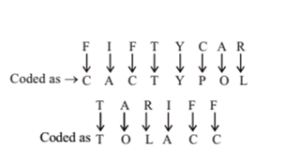# RRB ALP 2018 Practice Test Papers | Reasoning Questions (Day-31)

Dear Aspirants, Here we have given the Important RRB ALP & Technicians Exam 2018 Practice Test Papers. Candidates those who are preparing for RRB ALP 2018 can practice these Reasoning Questions to get more confidence to Crack RRB 2018 Examination.

[WpProQuiz 2007]

Click “Start Quiz” to attend these Questions and view Solutions

1. Three of the following four are alike in a certain way and so form a group. Which is the one that does not belong to that group?

(a) 29

(b) 85

(c) 147

(d) 125

1. 10, 15, 24, 35, 54, 75, 100

(a) 35

(b) 75

(c) 24

(d) 15

1. 1, 3, 4, 7, 11, 18, 27, 47

(a) 27

(b) 11

(c) 18

(d) 7

1. Q travels towards East. M travels towards North. S and T travel in opposite directions. T travels towards right of Q. Which of the following is definitely true?

(a) M and S travel in the opposite directions.

(b) S travels towards West.

(c) T travels towards North.

(d) M and S travel in the same direction.

1. If ‘+’ means ‘minus’ ‘–’ means ‘multiplied by’ ‘¸’ means ‘plus’ and ‘×’ means ‘divided by’, then 10 × 5 ¸ 3 – 2 + 3 =?

(a) 5

(b) 21

(c) 53/ 3

(d) 18

1. In a language FIFTY is written as CACTY, CAR as POL, TAR as TOL, how can TARIFF be written in that language?

(a) TOEFEL

(b) TOEFDD

(d) TOLACC

1. Some equations have been solved on the basis of certain system. Find the correct answer for the unsolved equation on that basis. If 94 + 16 = 42, 89 + 23 = 78, then 63 + 45 =?

(a) 18

(b) 28

(c) 38

(d) 48

1. Two positions of a dice are shown below. If 1 is at the bottom, which number will be on top?(a) 4

(b) 3

(c) 8

(d) 5

1. Read the figure and find the region representing persons who are educated and employed but not confirmed.(a) abc

(b) bd

(d) ac

1. In a certain code language BEAM is written as 5% * K and COME is written as \$7 K%. How is BOMB written in that code?

(a) 5% K5

(b) 5 7 K5

(c) \$7 K \$

(d) 5\$% 5

All other numbers are in the form of n2+4 where n is a natural number.

The series is +5, +9, +13, +17…. The difference in successive nos. 9 – 5 = 13 – 9 = 17 – 13 =…. = 4. Hence, 35 is wrong. It should be 37.

The sum of the first two nos. is the third no. Hence, 27 is wrong. It should be 29.

We have been given that Q travels towards East and M travels towards North. Now, T travels towards right of Q implies that T travels towards South. Hence, S travels towards North (because S and T travel in opposite directions). Therefore, it is definitely true that M and S travel in the same direction i.e., North.

1. Answer: (a)2. Answer: (d)9 × 4 + 1 × 6 = 36 + 6 = 42

8 × 9 + 2 × 3 = 72 + 6 = 78

Similarly 6 × 3 + 4 × 5 = 18 + 20 = 38

By looking, the dice position, we can say that 2, 4, 5 and 6 are adjacent faces of 3. therefore, if 1 number is at the bottom then 3 will be on the top.

The letters ‘b’ and ‘d’ are present in both the circles.

Here, B=> 5, E=>%, A => *, M=> K, C=> \$,O=> 7 Therefore, BOMB=> 57K5

RRB ALP 2018 Practice Test Papers | Reasoning Questions (Day-1)

RRB ALP 2018 Practice Test Papers | Reasoning Questions (Day-2)

RRB ALP 2018 Practice Test Papers | Reasoning Questions (Day-3)

RRB ALP 2018 Practice Test Papers | Reasoning Questions (Day-4)

RRB ALP 2018 Practice Test Papers | Reasoning Questions (Day-5)

RRB ALP 2018 Practice Test Papers | Reasoning Questions (Day-6)

RRB ALP 2018 Practice Test Papers | Reasoning Questions (Day-7)

RRB ALP 2018 Practice Test Papers | Reasoning Questions (Day-8)

RRB ALP 2018 Practice Test Papers | Reasoning Questions (Day-9)

RRB ALP 2018 Practice Test Papers | Reasoning Questions (Day-10)

RRB ALP 2018 Practice Test Papers | Reasoning Questions (Day-11)

RRB ALP 2018 Practice Test Papers | Reasoning Questions (Day-12)

RRB ALP 2018 Practice Test Papers | Reasoning Questions (Day-13)

RRB ALP 2018 Practice Test Papers | Reasoning Questions (Day-14)

RRB ALP 2018 Practice Test Papers | Reasoning Questions (Day-15)

RRB ALP 2018 Practice Test Papers | Reasoning Questions (Day-16)

RRB ALP 2018 Practice Test Papers | Reasoning Questions (Day-17)

RRB ALP 2018 Practice Test Papers | Reasoning Questions (Day-18)

RRB ALP 2018 Practice Test Papers | Reasoning Questions (Day-19)

RRB ALP 2018 Practice Test Papers | Reasoning Questions (Day-20)

RRB ALP 2018 Practice Test Papers | Reasoning Questions (Day-21)

RRB ALP 2018 Practice Test Papers | Reasoning Questions (Day-22)

RRB ALP 2018 Practice Test Papers | Reasoning Questions (Day-23)

RRB ALP 2018 Practice Test Papers | Reasoning Questions (Day-24)

RRB ALP 2018 Practice Test Papers | Reasoning Questions (Day-25)

RRB ALP 2018 Practice Test Papers | Reasoning Questions (Day-26)

RRB ALP 2018 Practice Test Papers | Reasoning Questions (Day-27)

RRB ALP 2018 Practice Test Papers | Reasoning Questions (Day-28)

RRB ALP 2018 Practice Test Papers | Reasoning Questions (Day-29)

RRB ALP 2018 Practice Test Papers | Reasoning Questions (Day-30)